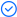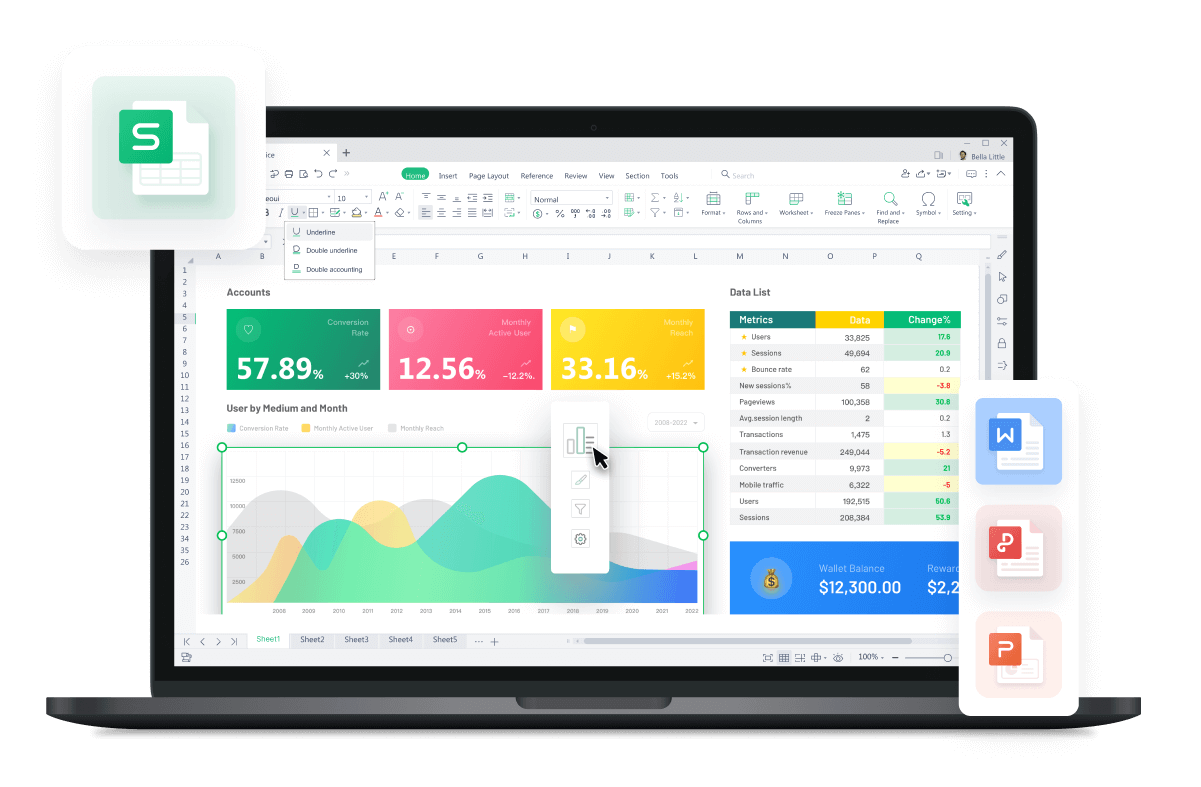WPS Office

Free All-in-One Office Suite with PDF EditorRead, edit, and convert PDFs with the powerful PDF toolkit.Microsoft-like interface, easy to use.

Windows • MacOS • Linux • iOS • Android# Top 10 leading excel Sum Formula templates

August 30, 2022
2.9K Views

Excel SUM is one of the most common formula of free sheet template. It has different ways to use. Its function is basically to total values in ranges of cells. Its function is to add values. Simply add values individually on WPS as it’s the most efficient website for using Excel Sum Formula Templates . It will manage data in sequence and understandable manner.

Household Balance Sheet:-

Household balanced sheet shows aggregate household wealth by value of assets of household. Here are sum of income and expenses of one year of household add on spreadsheet using Excel sum formula.

Shopping List with Calculator:-

The above mention is the Excel Sum formula template that shows the sum total of various items with respect to there amount of purchased.

Formula Statistic Statement Of Personnel Of Each Department:-

Excel Sum formula helpful to total each department personnel. How many male, female, college above, married, unmarried and headcounts are working in each department.

Store October Merchandise Sales Monthly Report:-

By using Excel Sum Formula you can easily compare sales of current month with previous month. Its very convenient to use and add data to get sum of sales each month.

Sales Performance Report:-

By Excel Sum formula you can easily manage you sellers per month. Excel Sum Formula is helpful to sum the total sales each month by each seller and keep tracking performance.

Financial Monthly Budget Statement:

By help of Excel Sum Formula you can sum up all budgets of various things used monthly like, it helps to manage budget of , electricity, rent etc.

Quarterly Budget Expenditure Statistical Report:-

It shows evaluation of different departments. It shows budget implementation rates. All of these sum of Budgets and Spending are obtained by using Excel Sum Formula.

Financial Reception Budget:

This one relates with Financial Reception Budget. By using Excel Sun Formula you can add Budgets for different purposes.

Second Half of Each Department Financial Expenditure:-

Simply using Excel Sum Formula, you can add expenditure on monthly  basis. Also it allows to compare departments along with percentages. Also  shows expenditure budgets for second half year.

Green product sales table:-

Above mentioned show sales of different products in three months using Excel Sum Formula. Through this we can evaluate sales in total with pi-charts help.

WPS Office is an appropriate website to download free templates of free spreadsheet on which by using Excel Sum Formula Templates you can do many calculations. WPS Office give free templates and you should download WPS office to manage your yearly or monthly or daily base data.